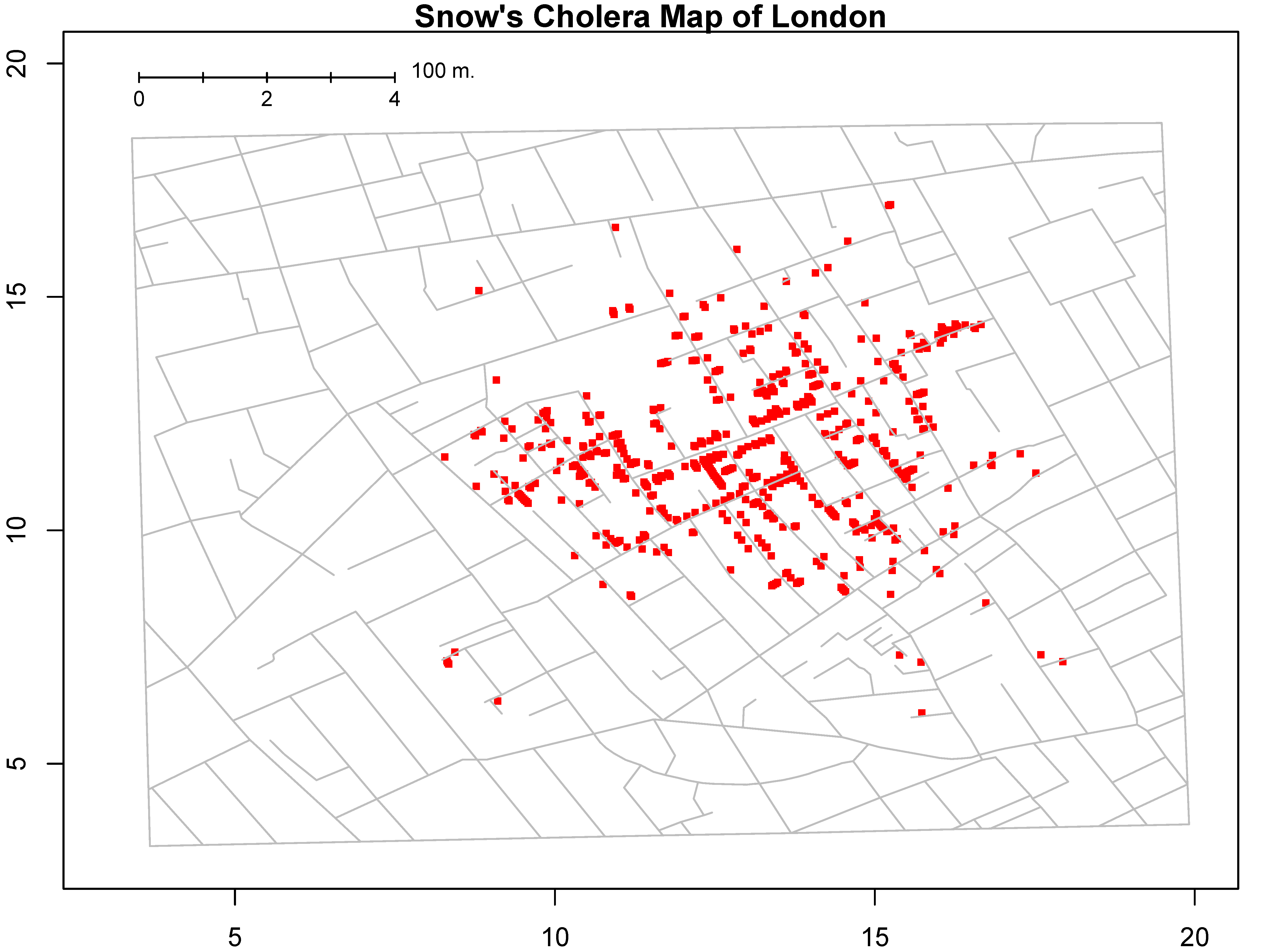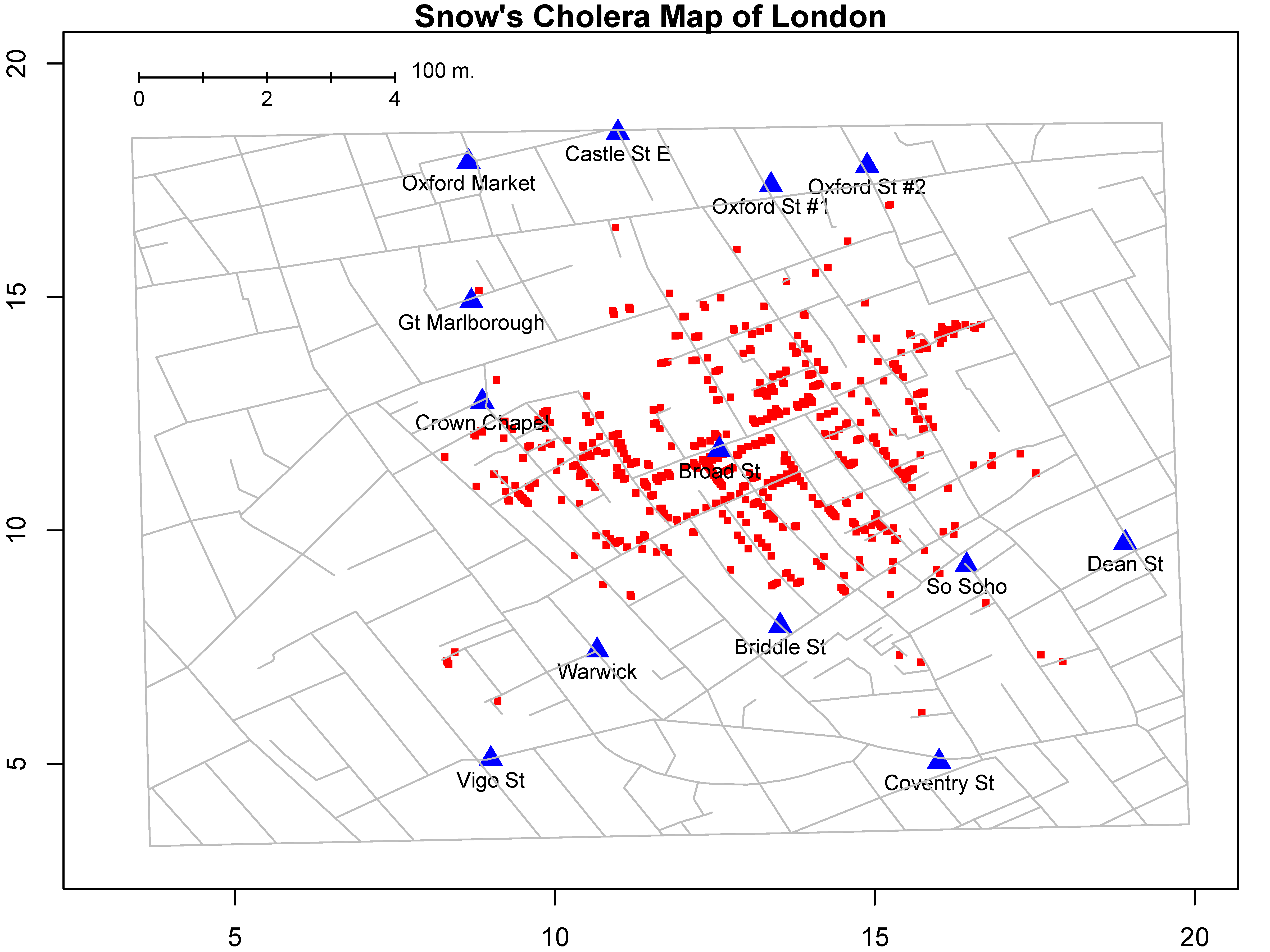# 第 4 章 数据可视化

library(tidyverse)
library(patchwork)

## 4.1 为什么要可视化## 4.2 ggplot2 的图形语法

• 数据 (data)
• 映射 (mapping)
• 几何对象 (geom)
• 统计变换 (stats)
• 标度 (scale)
• 坐标系 (coord)
• 分面 (facet)
• 主题 (theme)
• 存储和输出 (output)

mpg %>% select(displ, hwy, cyl, class)
• displ： 发动机排量
• hwy： 每加仑英里数
• cyl：汽缸数目
• class：汽车类型

ggplot(data = mpg, aes(x = displ, y = hwy)) +
geom_point()
• ggplot()表示调用该函数画图，data = mpg 表示使用mpg数据框来画图。
• aes()表示数据和视觉属性之间的映射，通俗来讲，不同的数值，转换成不同的位置、色彩或透明度等。

aes(x = displ, y = hwy)，意思是变量displ作为（映射为）x轴方向的位置，变量hwy作为（映射为）y轴方向的位置。 - +表示添加图层。 - geom_point()表示绘制散点图。ggplot(data = mpg, aes(x = displ, y = hwy, color = class)) +
geom_point()ggplot(data = mpg, aes(x = displ, y = hwy, size = class)) +
geom_point()

ggplot(data = mpg, aes(x = displ, y = hwy, shape = class)) +
geom_point()

ggplot(data = mpg, aes(x = displ, y = hwy, aphha = class)) +
geom_point()

## 4.3 映射 vs.设置

ggplot(mpg, aes(displ, hwy)) + geom_point(color = "blue")ggplot(mpg, aes(displ, hwy)) + geom_point(size = 5)
ggplot(mpg, aes(displ, hwy)) + geom_point(shape = 3)
ggplot(mpg, aes(displ, hwy)) + geom_point(alpha = 0.5)

ggplot(mpg, aes(x = displ, y = hwy, color = "blue")) +
geom_point()## 4.4 几何对象

geom_point() 可以画散点图，也可以使用geom_smooth()绘制平滑曲线，

p1 <- ggplot(data = mpg, aes(x = displ, y = hwy)) +
geom_point()

p2 <- ggplot(data = mpg, aes(x = displ, y = hwy)) +
geom_smooth()

p3 <- ggplot(data = mpg, aes(x = displ, y = hwy)) +
geom_point() +
geom_smooth()

p1 + p2 + p3## 4.5 Global vs. Local

ggplot(mpg, aes(x = displ, y = hwy, color = class)) +
geom_point()ggplot(mpg) +
geom_point( aes(x = displ, y = hwy, color = class))ggplot(mpg, aes(x = displ, y = hwy, color = class)) +
geom_point()

x = displ, y = hwy, color = class 为全局变量。如果映射关系aes() 写在几何对象geom_point()里, 就为局部变量, 比如。

ggplot(mpg) +
geom_point(aes(displ, hwy, color = class))

ggplot(mpg, aes(x = displ, y = hwy, color = class)) +
geom_point() +
geom_smooth()

ggplot(mpg, aes(x = displ, y = hwy, color = class)) +
geom_point(aes(color = factor(cyl)))ggplot(mpg, aes(displ, hwy, color = class)) +
geom_smooth(method = lm) +
geom_point()ggplot(mpg, aes(displ, hwy)) +
geom_smooth(method = lm) +
geom_point(aes(color = class))## 4.6 saving plots

ggsave("myfirst_plot.pdf", width = 6, height = 6, dpi = 300)